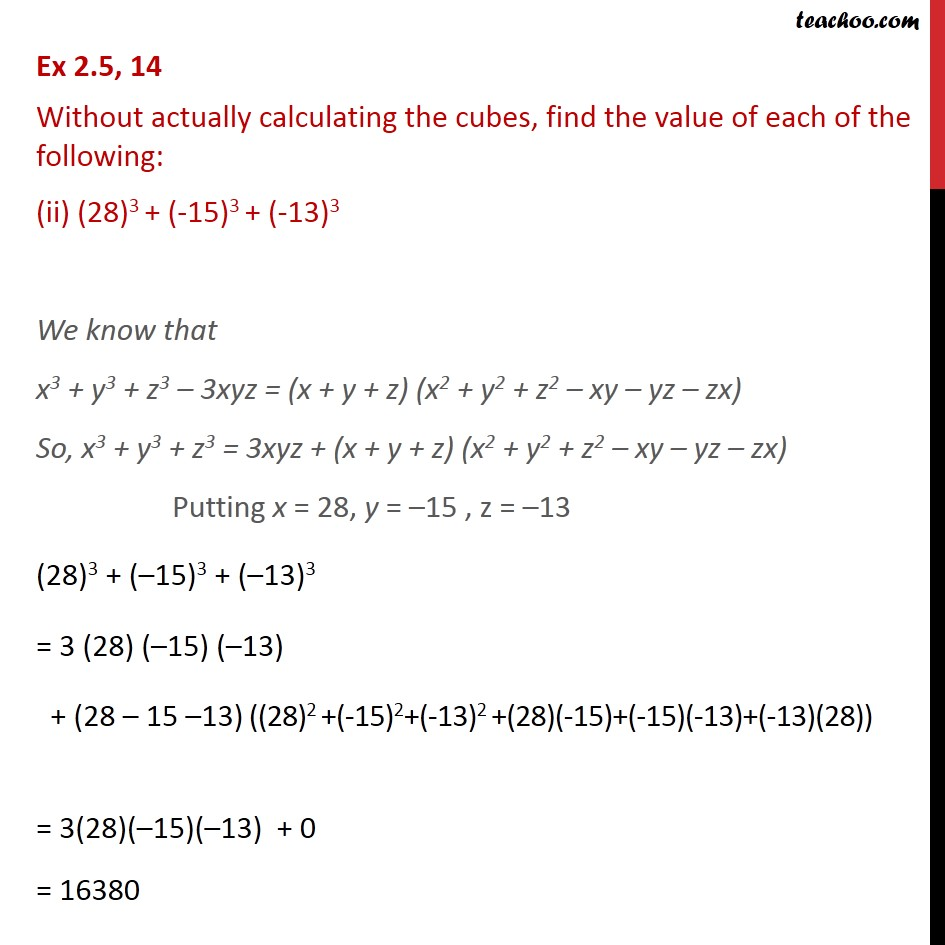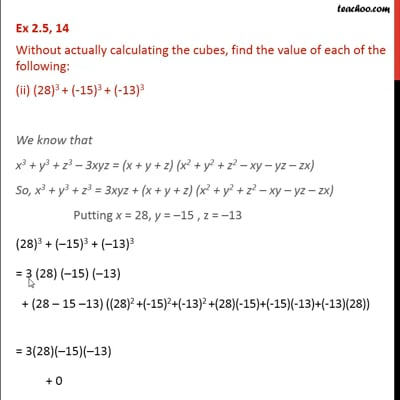Ex 2.5

Chapter 2 Class 9 Polynomials
Serial order wiseThis video is only available for Teachoo black users

Maths Crash Course - Live lectures + all videos + Real time Doubt solving!

### Transcript

Ex 2.5, 14 Without actually calculating the cubes, find the value of each of the following: (ii) (28)3 + (-15)3 + (-13)3 We know that x3 + y3 + z3 3xyz = (x + y + z) (x2 + y2 + z2 xy yz zx) So, x3 + y3 + z3 = 3xyz + (x + y + z) (x2 + y2 + z2 xy yz zx) Putting x = 28, y = 15 , z = 13 (28)3 + ( 15)3 + ( 13)3 = 3 (28) ( 15) ( 13) + (28 15 13) ((28)2 +(-15)2+(-13)2 +(28)(-15)+(-15)(-13)+(-13)(28)) = 3(28)( 15)( 13) + 0 = 16380